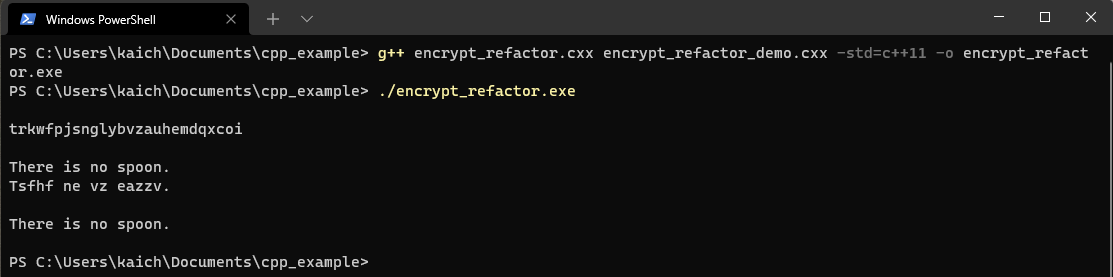# 單元 22 - 重構Source code → Refactoring
Maintainability
Extensibility

5 × 10 = 50

1 × 2 × 3 × ⋯⋯ × 25 × 26 = 4.0329 × 1026

```// 宣告 Encrypt 類別
class Encrypt {
public:
// 宣告建構函數
Encrypt(string s = "");
// 宣告 setter 成員函數
void set_code_array(string);
// 宣告 getter 成員函數
string get_code_array();
// 宣告編碼、解碼的成員函數
string ToEncode(string);
string ToDecode(string);

private:
// 密碼表字串
string code_array;
// 字母表字串
string alphabet_array;
};```

```// 宣告建構函數
Encrypt(string s = "");```

```// 字母表字串
string alphabet_array;```

```// Encrypt 的建構函數
Encrypt::Encrypt(string s) {
// 建立字母表字串
alphabet_array = "abcdefghijklmnopqrstuvwxyz";

// 判斷是否由參數設定密碼表
if (s != "") {
code_array = s;
}
else {
// 初始化密碼表字串
code_array = "abcdefghijklmnopqrstuvwxyz";
// 獲取時鐘週期個數
unsigned int seed = system_clock::now().time_since_epoch().count();
// 攪亂字串中的元素順序
shuffle(code_array.begin(), code_array.end(), mt19937(seed));
}
}```

```// 建立字母表字串
alphabet_array = "abcdefghijklmnopqrstuvwxyz";```

```// 判斷是否由參數設定密碼表
if (s != "") {
code_array = s;
}```

```else {
// 初始化密碼表字串
code_array = "abcdefghijklmnopqrstuvwxyz";
// 獲取時鐘週期個數
unsigned int seed = system_clock::now().time_since_epoch().count();
// 攪亂整數陣列中的元素順序
shuffle(code_array.begin(), code_array.end(), mt19937(seed));
}```

```// 進行編碼工作的成員函數
string Encrypt::ToEncode(string s) {
// 由參數字串取得字元的暫存變數
char c;
// 暫存編碼結果的字串
string r;
// 利用迴圈走完參數字串的所有字元
for (char c: s) {
// 判斷該字元是否為英文小寫字母，若是英文小寫字母就進行編碼轉換
if (islower(c)) {
r += alphabet_array.at(code_array.find(c));
}
else {
r += c;
}
}

// 結束回傳編碼過的字串
return r;
}```

```// 利用迴圈走完參數字串的所有字元
for (char c: s) {
// 判斷該字元是否為英文小寫字母，若是英文小寫字母就進行編碼轉換
if (islower(c)) {
r += code_array.at(alphabet_array.find(c));
}
else {
r += c;
}
}```

`for (char c: s) {`

`if (islower(c)) {`

islower() 在標準程式庫 cctype 中，這是個布林函數 (Boolean function) ，也就是如果參數是英文小寫字母就回傳 true ，否則回傳 false

`r += code_array.at(alphabet_array.find(c));`

```else {
r += c;
}```

```// 引入 Encrypt 類別的標頭檔
#include "encrypt_refactor.h"```GUI 篇與會使用重構版的 Encrypt 類別當作計算核心，在此之前，我們先來好好認識一下標準程式庫囉！

1. 重構是指重新整理程式碼，讓程式更易於維護。
2. 常見的重構技術包括整理資料成員、成員函數，挑出類別的共通特性定義父類別等等。
3. Encrypt 類別的重構主要分成兩大項目，其一為建立密碼表改用標準程式庫攪亂字串順序的程式，其二為編碼、解碼改用索引值對照的方式。

1. 什麼是重構？為什麼要替開發好的程式進行重構？
2. Encrypt 類別進行了哪些重構？為什麼要作這些重構？

1. main() 函數的小括弧中可以宣告整數參數，如 int argc ，以及字元陣列的指標參數，如 char *argv[] ，這樣可以取得命令列參數，也就是執行程式後的依空格格開的文字會依序儲存在 argv[] 內， argc 則是數量，寫一個程式 exercise2201.cxx ，加入取得命令列參數的兩個參數，然後用迴圈印出命令列參數。 參考程式碼
2. 承上題，寫一個程式 exercise2202.cxx ，假設命令列參數輸入都是整數，然後利用迴圈計算命令列參數的輸入總和。 參考程式碼
3. 標準程式庫中 cstdlibsystem() 可用於呼叫系統指令，這裡的指令須以字串代入，寫一個程式 exercise2203.cxx ，利用 system() 呼叫 "man ls"man 在 Linux 系統中用來查詢指令手冊，因此 man ls 是查詢 ls 指令的用法。 參考程式碼
4. 承上題，標準程式庫中 unistdchdir() 可用於切換工作路徑，路徑參數須以字串代入，寫一個程式 exercise2204.cxx ，先用 Linux 指令 mkdir 建立 demo 目錄，然後 chdir() 進入這個目錄，然後用 touch 建立 demo.txt 檔案，再用 ls 印出此目錄下的檔案列表。 參考程式碼
5. 承上題，寫一個程式 exercise2205.cxx ，繼續利用 Linux 指令 mkdirdemo 內建立 demo 目錄，然後用指令 cpdemo.txt 複製到新路徑。 參考程式碼
6. 承上題，寫一個程式 exercise2206.cxx ，改用 Linux 指令 mvdemo.txt 移動到新路徑，比較 cpmv 的差異。 參考程式碼
7. 承上題，寫一個程式 exercise2207.cxx ，利用 Linux 指令 date 印出現在時間。 參考程式碼
8. 承上題，寫一個程式 exercise2208.cxx ，利用 Linux 指令 cal 印出現在的月曆。 參考程式碼
9. 承上題，寫一個程式 exercise2209.cxx ，利用 Linux 指令 more 顯示程式檔案內容。 參考程式碼
10. 承上題，寫一個程式 exercise2210.cxx ，利用 Linux 指令 pwd 顯示目前所在路徑。 參考程式碼